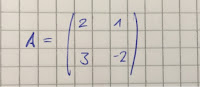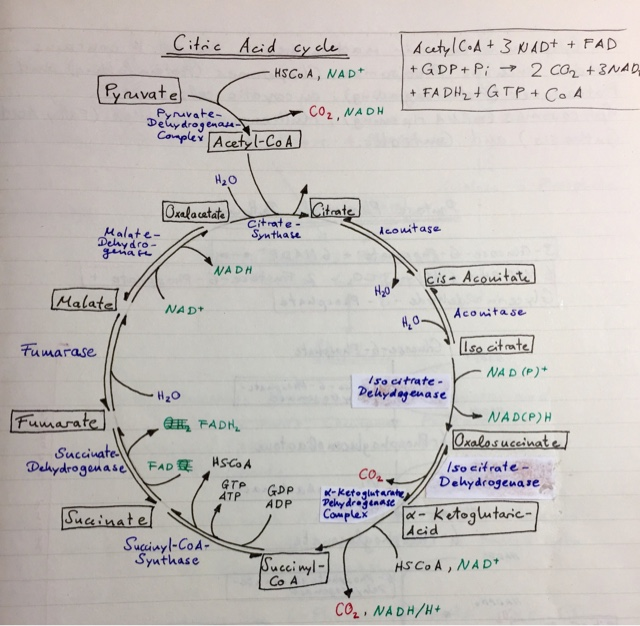Posts

Showing posts from April, 2017

How To - Determinants of MatricesThe determinant of a matrix is very useful and commonly asked for in exams. With it, you can determine if a matrix is invertible (thus find the inverse for sure, without wasting tons of precious time in an exam) and it's a sure way to get points even if you are pretty hopeless and just want to pass

There are three basic cases for matrices, dimensions 2x2, 3x3 and dimensions 3<.
Results for the three exercises will be posted at the end.

Tipp: a matrix can only be inverted if the determinant does not equal 0 !

In the case of matrix A (2x2) the main- minus minor-diagonal formula applies; with upper left times lower right, minus upper right times lower left.

For example B (3x3) it becomes more complicated. We can apply the rule of Sarrus (Wikipedia). For that, we replicate the first two columns to the right side. Now we create the main and minor diagonals, as seen in the picture below:

After this, we subtract the sum of the minors from the sum of the main diagonals and receive the determ…

How To - Citric Acid CycleThe citric acid cycle is one of the central logistical hub in our metabolism. It provides oxidative breakdown for organic substances, energy by releasing CO2 and H2O from Acetyl-CoA and so on.

There are 10 basic steps to the cycle and its goal is to catalyze the condensation of Oxalacetate to Citrate.
I will list the intermediates of this reaction and their respective enzymes, as well as their components:
Citrate + Aconitase -> cis-Aconitate  (H20)
cis-Aconitate + Aconitase -> Isocitrate(H20)
Isocitrate + Isocitrate-dehydrogenase -> Oxalsuccinate (NAD+, NADH)
Oxalsuccinate + Isocitrate-dehydrogenase -> Alpha-ketoglutaric-acid  (CO2)
Alpha-ketoglutaric-acid + alpha-ketoglutarate-dehydrogenase-complex -> Succinyl-CoA  (CoA, NAD+, CO2, NADH/H+)
Succinyl-CoA + Succinyl-CoA-Synthase -> Succinate (GDP, ADP, GTP, ATP)
Succinate + Succinate-dehydrogenase -> Fumarate  (FAD, FADH2)
Fumarate + Fumarase -> Malate  (H2O)
Malate + Malate-dehydrogenase -> Oxalacetate  (NAD+, NADH)
Oxa…